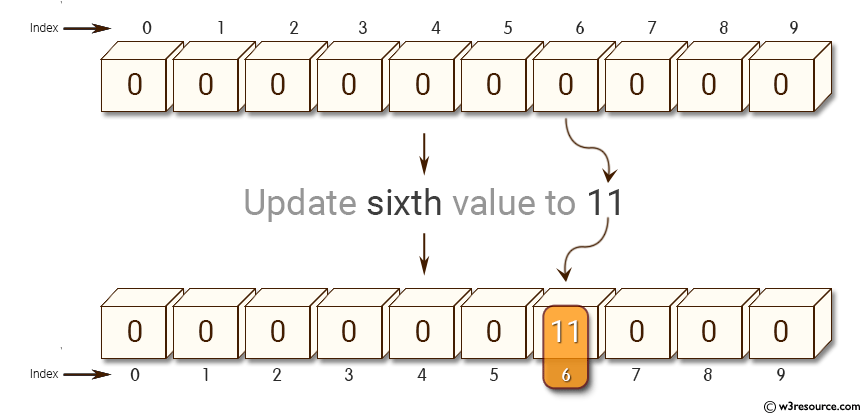﻿ NumPy: Create a null vector of size 10 and update sixth value to 11 - w3resource# NumPy: Create a null vector of size 10 and update sixth value to 11

## NumPy: Array Object Exercise-4 with Solution

Write a NumPy program to create a null vector of size 10 and update sixth value to 11.Sample Solution:-

Python Code:

``````
import numpy as np
x = np.zeros(10)
print(x)
print("Update sixth value to 11")
x = 11
print(x)
```
```

Sample Output:

```[ 0.  0.  0.  0.  0.  0.  0.  0.  0.  0.]
Update sixth value to 11
[  0.   0.   0.   0.   0.   0.  11.   0.   0.   0.]
```

Python Code Editor:

Have another way to solve this solution? Contribute your code (and comments) through Disqus.

What is the difficulty level of this exercise?

Test your Python skills with w3resource's quiz

﻿

## Python: Tips of the Day

Set comprehension:

```>>> m = {x ** 2 for x in range(5)}
>>> m
{0, 1, 4, 9, 16}
```